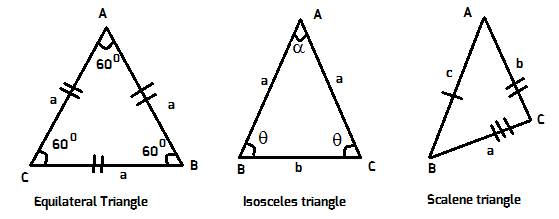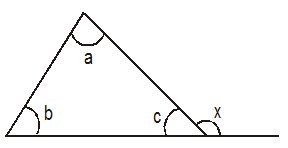# Types of Triangles and Similarity

## Types of triangles:

Triangles are classified based on their sides and based on the angles.
Based on Sides:1. Equilateral triangle: A triangle where all three sides are equal is called an equilateral triangle. Each angle in this triangle = ${60^0}$.  An equilateral triangle is also known as equi angles triangle.
2. Isosceles triangle: A triangle whose two and only two sides are equal is called an isosceles triangle. Two angles in this triangle are equal.
3. Scalene Triangle: A triangle whose all angles and sides are different is called Scalene triangle.

Based on Angles:1. Acute angle triangle: A triangle whose angles all less than 90 degrees is called acute angle triangle.
2. Obtuse angle triangle: A triangle which has an angle more than 90 degrees is called Obtuse angle triangle.
3. Right angle triangle: A triangle which has one angle equal to 90 degrees is called right-angle triangle.

External Angle of a triangle:The exterior angle $x$ is always equal to sum of the two remote internal angles. i.e.,$x = \angle a + \angle b$

## Similarity of triangles *: (V.Imp)

If two triangles are similar, their sides, their altitudes, their medians are in the same ratio. The most occur condition for similarity is $AAA$ similarity.

### AAA Similarity of the triangles*:If all the three angles of a triangle is equal to the corresponding three angles of the other triangle, then both the triangles are similar.
If, $\angle$A = $\angle$D, $\angle$B = $\angle$E, $\angle$C = $\angle$F, then $\Delta$ABC $\approx$ $\Delta$DEF
Note: While applying the $AAA$ similarity, always look for angles and their corresponding sides in two triangles in the same order.

### SSS similarity of triangles:

If all the three sides of a triangle is in proportion with the corresponding three sides of the other triangle, both the triangles are similar.
If, $\displaystyle\frac{{AB}}{{DE}} = \frac{{AC}}{{DF}} = \frac{{BC}}{{EF}}$, then $\Delta ABC \approx \Delta DEF$

### SAS condition of similarity:If the two sides of a triangle are in proportion with the corresponding two sides of the other triangle and the included angle of one is equal to the included angle of the other, the triangles are similar.
$\displaystyle\frac{{AB}}{{DE}} = \frac{{BC}}{{EF}}$ then $\angle B = \angle E$ then $\Delta ABC \approx \Delta DEF$